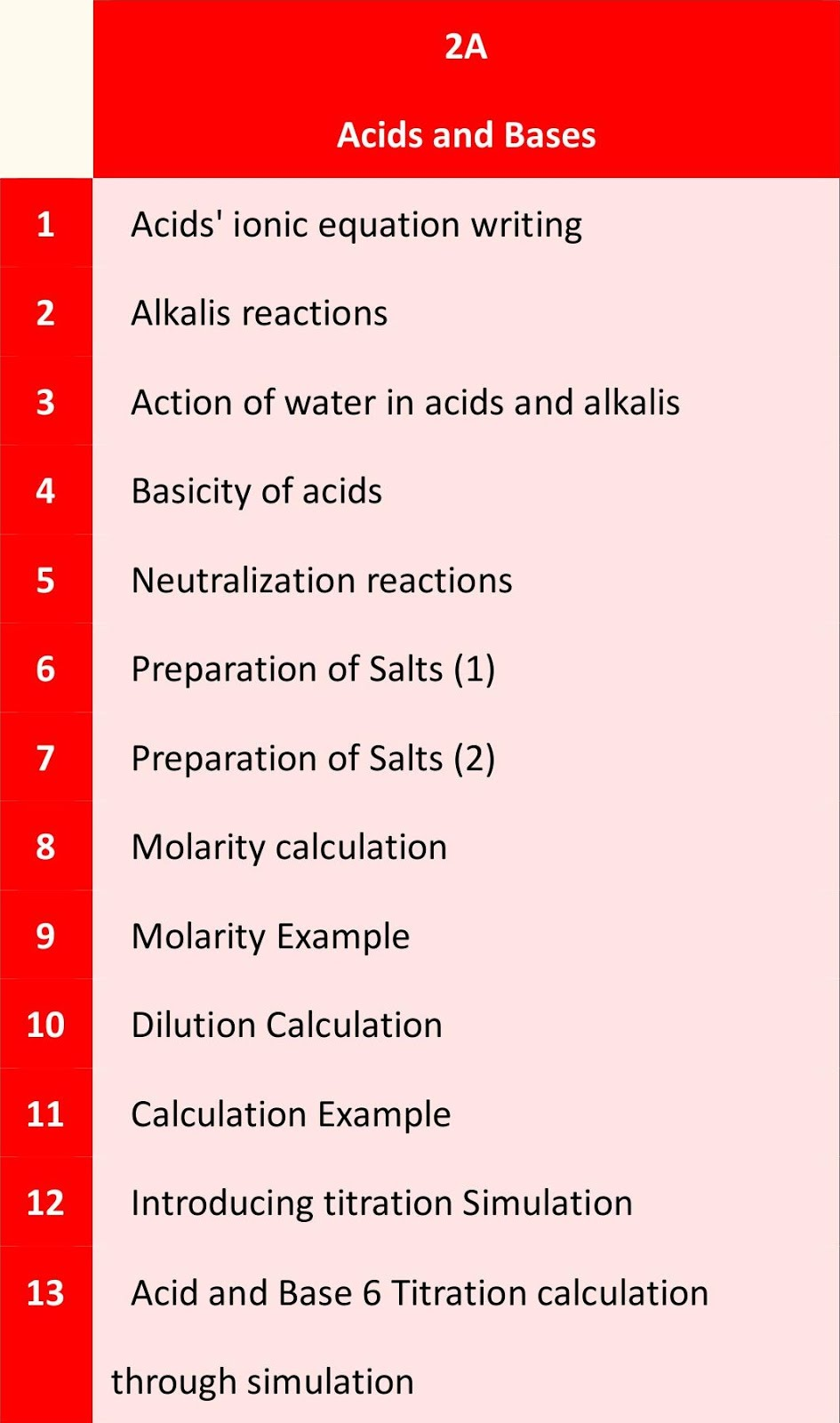Topic      1B         4A

2A        Acids and Bases1.          Acids' ionic equation writing

2.          Alkalis reactions

3.          Action of water in acids and alkalis

4.          Basicity of acids

5.          Neutralization reactions

6.          Preparation of Salts (1)

7.          Preparation of Salts (2)

8.          Molarity calculation

9.          Molarity Example

10.     Dilution Calculation

11.     Calculation Example

12.     Introducing titration Simulation

13.     Acid and Base 6 Titration calculation through simulation